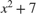# func2str

Construct character vector from function handle

## Syntax

``c = func2str(fh)``

## Description

example

````c = func2str(fh)` constructs a character vector, `c`, that contains the name of the function associated with the function handle, `fh`. If `fh` is associated with an anonymous function, `func2str` returns a character vector that represents the anonymous function.```

## Examples

collapse all

Create function handles for both the `cos` function and for an anonymous function, and then convert them to character vectors.

```fh = @cos; c = func2str(fh)```
```c = 'cos' ```
```fh = @(x,y)sqrt(x.^2+y.^2); c = func2str(fh); disp(['Anonymous function: ' c])```
```Anonymous function: @(x,y)sqrt(x.^2+y.^2) ```

Create a function that evaluates a function handle for a single input.

Create the following function in a file, `evaluateHandle.m`, in your working folder.

```function evaluateHandle(fh,x) y = fh(x); str = func2str(fh); disp('For input value: ') disp(x) disp(['The function ' str ' evaluates to:']) disp(y) end ```

Use a function handle to evaluate the `sin` function at `pi/2`.

```fh = @sin; x = pi/2; evaluateHandle(fh,x) ```
```For input value: 1.5708 The function sin evaluates to: 1 ```

Use a function handle to evaluatefor the specified matrix, `A`.

```fh = @(x) x.^2+7; A = [1 2;0 1]; evaluateHandle(fh,A) ```
```For input value: 1 2 0 1 The function @(x)x.^2+7 evaluates to: 8 11 7 8 ```

## Input Arguments

collapse all

Handle to convert to a character vector, specified as a function handle.

## Tips

• You lose variables stored in the function handle when you convert it to a character vector using `func2str`, and then back to a handle using `str2func`.

## Version History

Introduced before R2006a